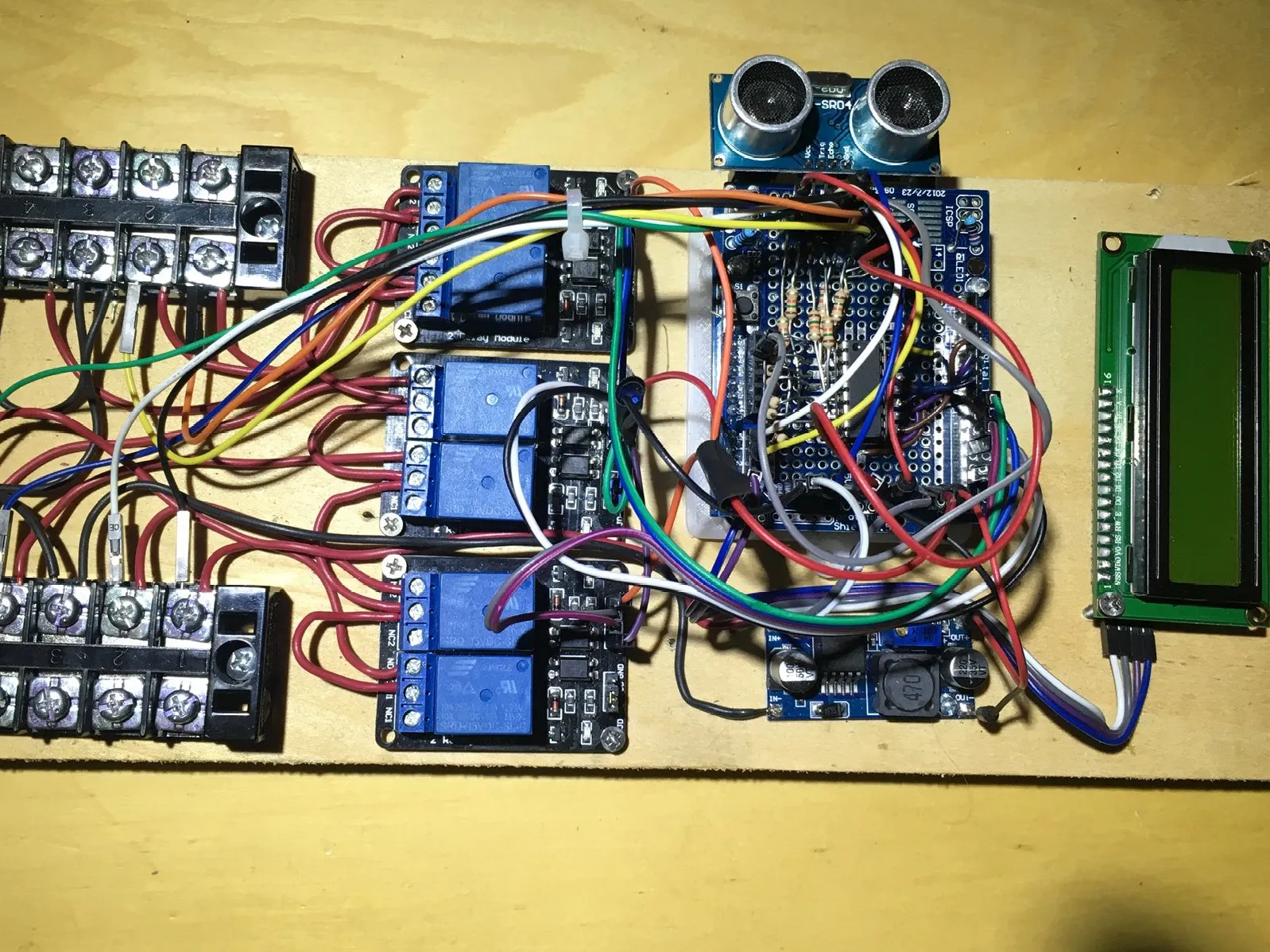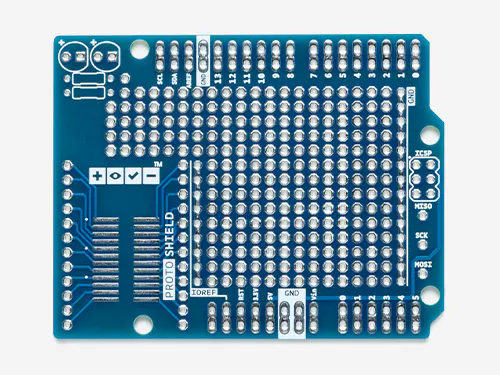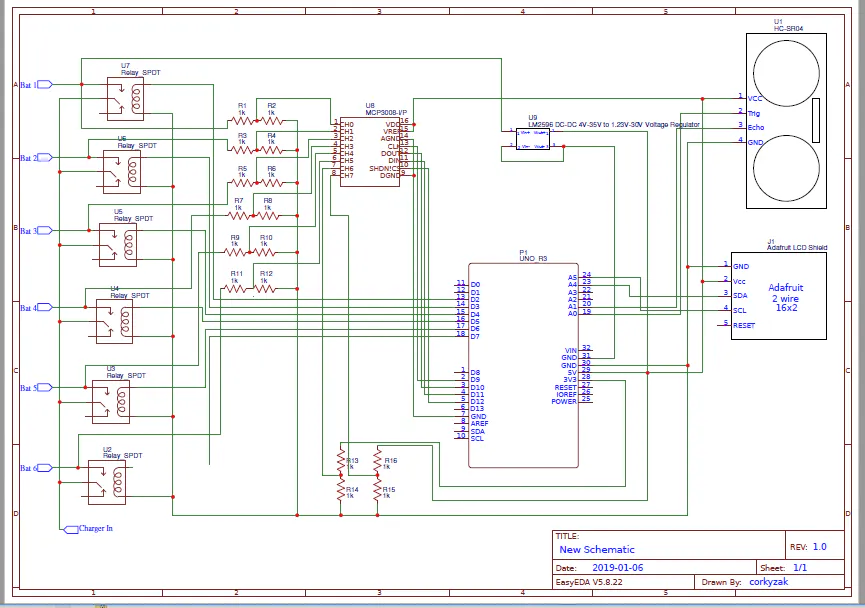# Smart Battery Charger Multiplexer with Smart Display

Use a smart battery charger on up to 6 batteries - wave your hand to turn display on and check charging.

IntermediateFull instructions provided4,378## Things used in this project

### Hardware componentsArduino UNO & Genuino UNO
×1Arduino Proto Shield
×1Ultrasonic Sensor - HC-SR04 (Generic)
×1
×1
 5V Relay - 2 channel optocoupler
×3
 LM2596 Power Supply Module DC / DC BUCK 3A adjustable
×1Resistor 1k ohm
×12
 6 Position Terminal Strip Check the picture - most any type of connection system can be used.
×1

### Hand tools and fabrication machinesSoldering iron (generic)

## Schematics

### Battery Multiplexer Schematic

Schematic for multiplexer## Code

### Battery Multiplexer Arduino Code

Arduino
I used an Arduino Uno.
```#include <Wire.h>
#include <LiquidCrystal_I2C.h> // Using version 1.2.1
#include <timer.h>
#include <HCSR04.h>

auto timer = timer_create_default(); // create a timer with default settings

// The LCD constructor - address shown is 0x27 - may or may not be correct for yours
// Also based on YWRobot LCM1602 IIC V1
LiquidCrystal_I2C lcd(0x27, 2, 1, 0, 4, 5, 6, 7, 3, POSITIVE);

int zcnt = 0;
int acnt = 0; // general purpuse right now
int chargeTime = (10 * 1000); // how much time to spend on each battery
int charge_time_counter = 59; // how many chargetimes were passed?
int charge_time_max = 60; // how many chargetimes were passed?
int relay1 = 3; // each relay on it's own pin
int relay2 = 4;
int relay3 = 5;
int relay4 = 6;
int current_relay = 0; // which relay is the one on now?
int max_relays = 3; // how may relays - 0 based. Start expecting all three.
float volts1; // what's the voltage of each battery
float volts2;
float volts3;
int min_volts = 1; // what is the minimum voltage a batter voltage should be to be charged.
int volts_update_count = 5; // how often to update the voltage reading in seconds
int volts_update_counter = 0; // track the count of seconds gone by
int volts_update = (500);
int time_on_counter = 0; // counter for the display on/off
int time_on_count = 100; //seconds to be on
int heart_beat = 0; // flip this 0 to 1 to 0 to show the heart beat

UltraSonicDistanceSensor distanceSensor(11, 12);  // Initialize sensor that uses digital pins 13 and 12.
int distance;

int relays[] = { relay1, relay2, relay3 };
int is_live[] = { 0, 0, 0}; // 1 if live, 0 if not. I have only 3

int debug = 5; // set this to the level of debug msgs to go to the serial printer

void all_change_to(int which) { // change all relays to HIGH or LOW, you tell me which:)
digitalWrite(relay1, which);
digitalWrite(relay2, which);
digitalWrite(relay3, which);
}

void toggle_relays(int whichOne) {
lcd.setCursor(0, 0); // show which relay is the current one on
lcd.print("Relay on:");
lcd.print(current_relay + 1); // current_relay is zero based
all_change_to(HIGH); // turn all off first
digitalWrite(relays[current_relay], LOW); // and set the one that should be on on
acnt++; // just a counter to show me it's working
lcd.print("  ");
lcd.print(acnt);
}

void checkRelays() {
// move the current_relay to the next relay
// if we hit the max, start at 0
// while you're here, call toggle_relays to make sure we're not charge a blank or dead one
charge_time_counter++;
if (charge_time_counter >= charge_time_max) {
current_relay++;
if (current_relay >= max_relays) {
current_relay = 0;
}
toggle_relays(current_relay);
charge_time_counter = 0;
}
}

// reaad the distance of anything from the HC-S204
// if it's something within 80cm then turn the display on and reset the display on counter
// if the counter is reached then nothing is within 80cm so turn the display off
distance = distanceSensor.measureDistanceCm();
// uncomment these to see the actual distance measured
// Serial.print("Distance in CM: ");
// Serial.println(distance);
if (distance < 80) { // can be anything under 200 for my system uncomment above and check yours
lcd.backlight();   // turn display on
time_on_counter = 0;
} else { // maybe going off?
time_on_counter++;
if (time_on_counter > time_on_count) {
time_on_counter = 0;
lcd.noBacklight();
}
}
}

// read the volts at each battery input
// then if less then min_volts, there is nothing there worth charging, skip that connection
// this gets called over and over so if a wire gets knocked off or
// whatever it won't be in the charge loop
volts1 = (volts1 * 0.016);
lcd.setCursor(0, 1); lcd.print("                "); // clear the line
lcd.setCursor(11, 0);
lcd.print(volts1);
volts2 = (volts2 * 0.0164);
lcd.setCursor(4, 1);
lcd.print(volts2);
volts3 = (volts3 * 0.0166);
lcd.setCursor(11, 1);
lcd.print(volts3);
// now test the voltages. If less than 10, then let's assume dead/bad or no battery
// so take it out of rotation
// start by clearing all relays out
int temp_cnt = 0;
// set all arrays to 0 - that's off
relays = 0;
relays = 0;
relays = 0;
if (volts1 > min_volts) {
relays[temp_cnt] = relay1;  // relay 1 is good
temp_cnt++;
}
if (volts2 > min_volts) {
relays[temp_cnt] = relay2;  // relay 2 is good
temp_cnt++;
}
if (volts3 > min_volts) {
relays[temp_cnt] = relay3;  // relay 3 is good
temp_cnt++;
}
max_relays = temp_cnt;
// this is a heart bet on the lcd - just shows me it's running
lcd.setCursor(0, 1);
if (heart_beat == 1) {
lcd.print("<>");
heart_beat = 0;
} else {
lcd.print("><");
heart_beat = 1;
}
lcd.print(charge_time_counter);
}

void setup()
{
Serial.begin(19200);
Serial.println("Starting");
lcd.begin(16, 2); // sixteen characters across - 2 lines
lcd.backlight();
pinMode(relay1, OUTPUT);
pinMode(relay2, OUTPUT);
pinMode(relay3, OUTPUT);
all_change_to(HIGH);
// setup timers. 3 timers - the time to turn the charger on each battery,
// how often to show the voltage update
// and how often to check for distance read to turn on the display
read_show_volts(); // do the first time so we don't have to wait for the timer.
checkRelays();
timer.every(chargeTime, checkRelays);
}

void loop()
{
timer.tick(); // tick the timer
}
```

## Credits

### leonzak

0 projects • 5 followers